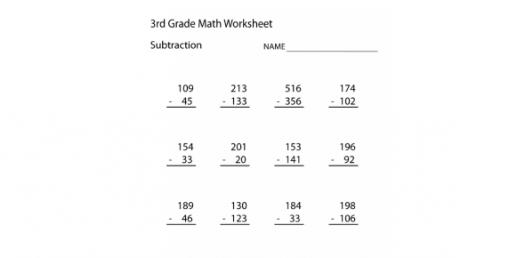# 3rd Grade Multiplication Quiz! Trivia Knowledge Test

20 Questions | Attempts: 508SettingsThis trivia knowledge test is a 3rd Grade Multiplication Quiz! It is perfect for testing out how attentive you have been in class and will give you an idea on how questions will be designed in your upcoming exams. If you feel like you need more practice, be sure to check out other quizzes just like it. All the best!

• 1.
3 X 7 =[Blank]
• 2.
4 X 8 =[Blank]
• 3.
5 X 8 =[Blank]
• 4.
6 X 4 =[Blank]
• 5.
7 X 7 =[Blank]
• 6.
9 X 2 =[Blank]
• 7.
5  x 10 =[Blank]
• 8.
9 X 3 =[Blank]
• 9.
8 X 6 =[Blank]
• 10.
3 X 5 =[Blank]
• 11.
9 X 4 =36
• A.

True

• B.

False

• 12.
6 X 6 =32
• A.

True

• B.

False

• 13.
5 X 7 = 35
• A.

True

• B.

False

• 14.
8 X 3 = 24
• A.

True

• B.

False

• 15.
2 X 7 =15
• A.

True

• B.

False

• 16.
3 X 7 =
• A.

24

• B.

21

• C.

18

• D.

14

• 17.
6 X 7 =
• A.

42

• B.

41

• C.

54

• D.

46

• 18.
8 X 5 =
• A.

45

• B.

40

• C.

35

• D.

30

• 19.
9 X 2 =
• A.

20

• B.

19

• C.

18

• D.

15

• 20.
2 X 7 =
• A.

15

• B.

16

• C.

20

• D.

14

## Related TopicsBack to top
×

Wait!
Here's an interesting quiz for you.